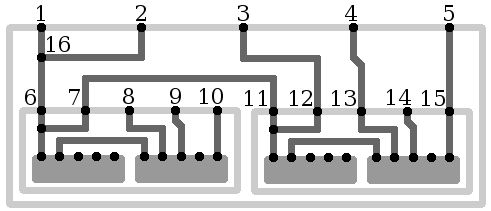시간 제한메모리 제한제출정답맞힌 사람정답 비율
1 초 (추가 시간 없음) 256 MB21150.000%

## 문제

In the layout of a recursive microcircuit, there is a total of $N$ contact points, with some pairs of contacts connected directly with conductive paths. In addition, there is a total of $S$ sub-circuits within the circuit, each being an exact copy of the circuit in question.

There are three types of contacts in the circuit layout:

1. Input contacts of the circuit ($K$ contacts). These are the only contacts connecting the circuit to external conductive paths.
2. Input contacts of the nested sub-circuits ($S \cdot K$ contacts).
3. Auxillary contacts.

All these contacts can be connected with each other by conductive paths without any restrictions.

Signals travel along conductive paths. When a signal reaches a contact, it can follow any of the paths connected to this contact. If an external signal reaches an input contact of the sub-circuit, it can enter the sub-circuit and travel further along its paths. If an internal signal reaches an input contact of the sub-circuit, it can leave the sub-circuit and travel outside the circuit (if there are paths outside, and if the external circuit is itself a sub-circuit of another circuit).

Consider the most external circuit, with nothing else on the outside. Determine whether two specified input contacts of the circuit are connected with paths. The contacts are connected if a signal can reach one contact from the other, possibly entering a number of various sub-circuits a finite number of times.

Apart from the fact of being connected, find out how deep the signal must go into the sub-circuits to reach one contact from the other. The external circuit has a nesting depth of 0; for its sub-circuits, the nesting depth is 1, and their sub-circuits, in turn, have a nesting depth of 2, etc. For an arbitrary path of the signal, the critical depth can be defined, which is the maximum nesting level among all circuits, whose conductive paths are travelled by the signal.

Determine the minimal value of the critical depth for the path between two given input contacts of the external circuit.

## 입력

The first line contains four integers: $N$ --- the number of contacts in the circuit layout, $K$ --- the number of input contacts of the circuit, $S$ --- the number of sub-circuits in the circuit, $M$ --- the number of conductive paths in the circuit layout ($1 \le K \le 100\,000$, $0 \le S \le 1\,000$, $K (S + 1) \le N \le 100\,000$, $0 \le M \le 100\,000$).

The following $M$ lines define the conductive paths in the  circuit layout. Each path is defined by two integers $a$ and $b$ --- the numbers of contacts in the layout directly connected by the path ($1 \le a \neq b \le N$).

The contacts in the circuit layout are numbered in numerical order from $1$ to $N$. The input contacts are numbered from $1$ to $K$. The input contacts of the $t$th sub-circuit are numbered from $t K + 1$ to $t K + K$ (for $1 \le t \le S$). The $j$th input contact on the $t$th circuit layout is the $(t K + j)$th contact on the external circuit layout. The remaining contacts, if such exist, are auxillary.

The following line contains an integer $Q$ --- the number of queries ($1 \le Q \le 100\,000$). Each of the remaining $Q$ lines contain one query that needs an answer. Each query is defined by two integers $u$ and $v$ --- the numbers of the input contacts of the external circuit ($1 \le u \neq v \le K$).

## 출력

In the output file, print $Q$ integers, one number per line. Every $r$th number must be an answer to the $r$th query: the nesting depth necessary to get from one of the input contacts to another. If there is no way to reach the other input contact, print the number $-1$ instead of the depth value.

## 예제 입력 1

16 5 2 7
1 16
2 16
6 16
7 11
3 12
4 13
5 15
4
1 2
2 3
3 4
4 5


## 예제 출력 1

0
1
2
-1


## 힌트